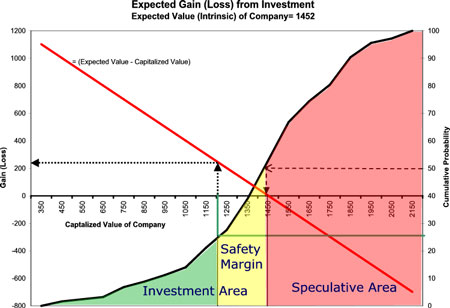# Risk, price and value

This entry is part 3 of 4 in the series A short presentation of S@R

Having arrived at the probability distribution for the value of equity (see full story) we are able to calculate expected gain, loss and their probability when investing in a company where the capitalized value (price) is known. (see “The Probability of Gain and Loss”)

In the figure below we have illustrated the investment and speculative area. The investment area comprice the part of the cumulative probability distribution below 50%.The speculative area is the area above 50%. The expected value is given at the 50% probability point (stapled line). The literature advices, and successful investors insists, on having a safety margin (discount) of at least 20% between expected value (intrinsic value) and the market price, as shown by the yellow area in the figure below. Graham and Dodd in Security Analysis introduced the concept of a margin of safety in 1934.

In a stochastic framework as ours it is better to set the safety margin at one of the percentiles or quartiles giving directly the value of the safety margin. A fixed percentage safety margin will always give a different probability for gain (loss), depending on the shape of the cumulative probability distribution.

An investor having a portfolio of stocks should thus use percentiles as a margin – having the same probability for gain (loss) throughout the portfolio. In the case below a 20% safety margin coincide with the first quartile, – giving a 25% probability for loss and 75% probability for gain. The expected value of the company is 1.452 the first quartile is 1.160 giving an exepcted gain of 292 or more with 75% probability (dotted lines).

We know that the total risk of any individual asset is the sum of the systematic and unsystematic risk. When computing the figure above we have used the company’s appropriate beta to account for the systematic risk (in calculating WACC). The unsystematic risk is given by the variance in the figure above.

In a well-diversified portfolio the expected value of the unsystematic return is assumed to be zero. When investing in a single asset we should be looking for assets with a high unsystematic return. In our context companies with a capitalized value below the percentile set as limit of the safety margin.

References

1. Security Analysis: The Classic 1934 Edition by Benjamin Graham, David L. Dodd. October 1, 1996, McGraw-Hill Professional Publishing; ISBN: 0070244960
2. and an interesting webiste The Graham-Buffett Teaching Endowment
Series Navigation<< The Case of Enterprise Risk ManagementThe Value of Information >>